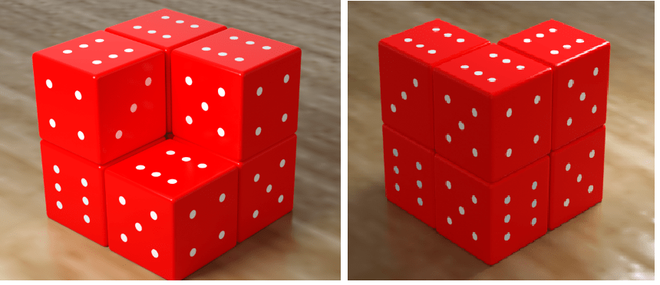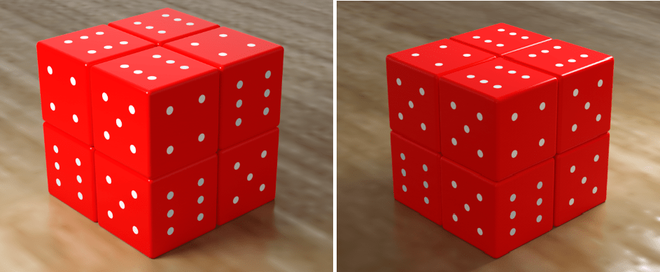# Check if max sum of visible faces of N dice is at least X or not

You are given N and an integer X, where N represents the number of dice. You have to arrange those dices in such a way that the visible number of faces are as minimum as possible and the sum of visible faces is as maximum as possible. The task is to check if the sum of values on the visible faces is at least X or not.

Approach: The problem is observation based and can be solved by using the Greedy Approach for getting the maximum possible sum. For more clarification follow the below illustration.

The problem can be solved by Greedy approach for getting maximum possible sum. We will see some cases below and will try to make a mathematical formula for calculating sum using some observations. Let us see some cases below:Structure of 1 dice

According to greedy approach for making maximum possible sum, We have to put 1 mark of dice on base surface, So that the visible faces will be 2, 3, 4, 5, 6. Maximum possible sum in this case will be 20.Structure of 2 dices

In structure of 2 dice, For making visible faces minimum as possible, We have to merge both dice, which will decrement a visible face of both dices and a face will be put as base. So that total 2 faces will be invisible for each dice. For maximizing sum, We have to chose those 2 invisible faces having minimum values, Which are 1 and 2. Visible faces of both dice will be 3, 4, 5 and 6. Total sum in this case = {3 + 4 + 5 + 6} + {3+ 4 + 5 + 6} = 18 + 18 = 36.Structure of 3 dices

For maximizing sum and minimizing visible number of faces, It is optimal to make three invisible faces(1, 2, 3) of one dice and two (1, 2) for two dices. For maximum sum we have to make invisible faces of lowest values first. Let us see the sum gained in this structure.

Format of Explanation is as:

Dice number: {Total faces of dice} – {Invisible faces} = {Visible faces} = Sum of visible faces
Dice 1: {1, 2, 3, 4, 5, 6} – {1, 2, 3}={4, 5, 6}=4 + 5 + 6 = 15
Dice 2: {1, 2, 3, 4, 5, 6} – {1, 2}={3, 4, 5, 6}=3 + 4 + 5 + 6 = 18
Dice 3: {1, 2, 3, 4, 5, 6} – {1, 2}={3, 4, 5, 6}=3 + 4 + 5 + 6 = 18

Total maximum sum=18 + 18 + 15 = 51. It can be verified that it is the maximum sum such that visible faces should be as minimum as possible.Struture of 4 dices

For minimizing visible faces, We can make at most 3 invisible faces for each dice, Same can be seen in the picture above. For maximizing sum, 1, 2 and 3 must be as invisible faces and 4, 5 and 6 should be visible faces of each dice. In this case, Maximum sum will be = {4, 5, 6} + {4, 5, 6} + {4, 5, 6} + {4, 5, 6} = 15 + 15 + 15 + 15 = 60, Which is maximum possible.Structure of 7 dices

Three dices at base level will have visible faces as 5 and 6 and one dice at base level will have 4, 5, 6 as visible faces. The arrangement of upper three dices 5th, 6th and 7th will follow the case of N = 3.

Level 1:
Dice 1: {1, 2, 3, 4, 5, 6} – {1, 2, 3, 4}={5, 6} = 5 + 6 = 11
Dice 2: {1, 2, 3, 4, 5, 6} – {1, 2, 3, 4} = {5, 6} = 5 + 6 = 11
Dice 3: {1, 2, 3, 4, 5, 6} – {1, 2, 3, 4} = {5, 6} = 5 + 6 = 11
Dice 4: {1, 2, 3, 4, 5, 6} – {1, 2, 3} = {4, 5, 6} = 4 + 5 + 6 = 15

Level 2:
Dice 5: {1, 2, 3, 4, 5, 6} – {1, 2} = {4, 5, 6} = 4 + 5 + 6 = 15
Dice 6: {1, 2, 3, 4, 5, 6} – {1, 2} = {3, 4, 5, 6} = 3+ 4 + 5 + 6 = 18
Dice 6: {1, 2, 3, 4, 5, 6} – {1, 2} = {3, 4, 5, 6} = 3 + 4 + 5 + 6 = 18
total maximum possible sum 11+ 11 + 11 + 15 + 15 + 18 + 18 = 99Structure of 8 dices

All the 4 dices present at level 1, Will have only 2 visible faces 5 and 6. While all the 4 dices at level 2 will have three visible faces 4, 5 and 6.

Level 1:
Dice 1: {1, 2, 3, 4, 5, 6} – {1, 2, 3, 4} = {5, 6} = 5 + 6 = 11
Dice 2: {1, 2, 3, 4, 5, 6} – {1, 2, 3, 4} = {5, 6} = 5 + 6 = 11
Dice 3: {1, 2, 3, 4, 5, 6} – {1, 2, 3, 4} = {5, 6} = 5 + 6 = 11
Dice 4: {1, 2, 3, 4, 5, 6} – {1, 2, 3, 4} = {5, 6} = 5 + 6 = 11

Level 2:
Dice 5: {1, 2, 3, 4, 5, 6} – {1, 2, 3} = {4, 5, 6} = 4 + 5 + 6 = 15
Dice 6: {1, 2, 3, 4, 5, 6} – {1, 2, 3} = {4, 5, 6} = 4 + 5 +6 = 15
Dice 7: {1, 2, 3, 4, 5, 6} – {1, 2, 3} = {4, 5, 6} = 4 + 5 + 6 = 15
Dice 8: {1, 2, 3, 4, 5, 6} – {1, 2, 3} = {4, 5, 6} = 4 + 5 + 6 = 15
Total maximum sum: 11 + 11 + 11 + 11 + 15+ 15 + 15 + 15 = 104

Observations: From the above observations, We can conclude some mathematical conditions for calculating maximum sum on N dices:

•  If N = 1, 2, 3, 4 then by following greedy approach maximum sum will be 20, 36, 51, 60 respectively
• Initialize ans = (N-4)*11
• If (N%4==0), ans += 15*4
• else If (N%4==1), ans+=15*3+20
• else If (N%4==2), ans+=15*2+18*2
• else, ans+=15*2+18*2

Below is the implementation of the above approach.